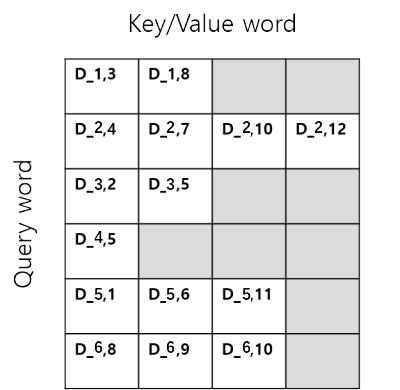# How can I do memory efficient attention with square mask

Hi, I wan to do attention calculation. For my task, from priori , I can block lots of query-key pair. (like Figure 1 and Figure 2) In Figure 1,2 , I paint gray for blocked query-key pair.

Figure 1. example of query-key pair tensor

Figure 2. example of query-key pair square mask

I use `mask` for attention calculation as below.

``````square_mask= (-1*) square_mask
attention_prob = nn.functional.softmax(attention_logit)
``````

I think that , computing in this way, even only a fraction of query-key pair what I should be really interested in, the amount of memory required to perform the calculation is still the same as using whole query-key pair.

As shown below(Figure 3&4), if we extract only the parts we need from the entire matrix and proceed with the calculation, I think that it can be an efficient calculation in terms of memory. How can we do this effectively?
Is there a better way other than this one?Figure 3. extracted query-key tensor

Figure 3. extracted query-key sqaure mask